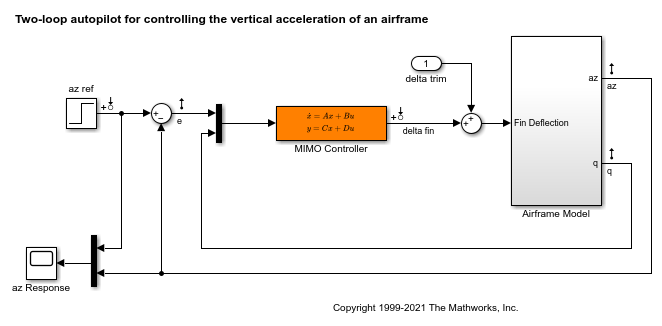# evalGoal

Evaluate tuning goals for tuned control system

## Syntax

``````[Hspec,fval] = evalGoal(Req,T)``````

## Description

example

``````[Hspec,fval] = evalGoal(Req,T)``` returns the normalized value `fval` of a tuning goal evaluated for a tuned control system `T`. The `evalGoal` command also returns the transfer function `Hspec` used to compute this value. ```

## Examples

collapse all

Tune a control system with `systune`, and evaluate the tuning goals with `evalGoal`.

Open the Simulink® model `rct_airframe2`.

```open_system('rct_airframe2') ```Create tracking, roll-off, stability margin, and disturbance rejection requirements for tuning the control system.

```Req1 = TuningGoal.Tracking('az ref','az',1); Req2 = TuningGoal.Gain('delta fin','delta fin',tf(25,[1 0])); Req3 = TuningGoal.Margins('delta fin',7,45); MaxGain = frd([2 200 200],[0.02 2 200]); Req4 = TuningGoal.Gain('delta fin','az',MaxGain); ```

Create an `slTuner` interface, and tune the model using these tuning goals.

```ST0 = slTuner('rct_airframe2','MIMO Controller'); rng default [ST1,fSoft] = systune(ST0,[Req1,Req2,Req3,Req4]); ```
```Final: Soft = 1.13, Hard = -Inf, Iterations = 95 ```

`ST1` is a tuned version of the `slTuner` interface to the control system. `ST1` contains the tuned values of the tunable parameters of the MIMO controller in the model.

Evaluate the margin goal for the tuned system.

```[hspec,fval] = evalGoal(Req3,ST1); fval ```
```fval = 0.5140 ```

The normalized value of the tuning goal is less than 1, indicating that the tuned system satisfies the margin requirement. For more information about how the normalized value of this tuning goal is calculated, see the `TuningGoal.Margins` reference page.

Evaluate the tracking goal for the tuned system.

```[hspec,fval] = evalGoal(Req1,ST1); fval ```
```fval = 1.1327 ```

The tracking requirement is nearly met, but the value exceeds 1, indicating a small violation. To further assess the violation, you can use `viewGoal` to visualize the requirement against the corresponding response of the tuned system.

## Input Arguments

collapse all

Tuning goal to evaluate, specified as a `TuningGoal` object or vector of `TuningGoal` objects. For a list of all `TuningGoal` objects, see Tuning Goals.

Tuned control system, specified as a generalized state-space (`genss`) model or an `slTuner` interface to a Simulink® model. `T` is typically the result of using the tuning goal to tune control system parameters with `systune`.

Example: `[T,fSoft,gHard] = systune(T0,SoftReq,HardReq)`, where `T0` is a tunable `genss` model

Example: `[T,fSoft,gHard] = systune(ST0,SoftReq,HardReq)`, where `ST0` is a `slTuner` interface object

## Output Arguments

collapse all

Transfer function associated with the tuning goal, returned as a state-space (`ss`) model. `evalGoal` uses `Hspec` to compute the evaluated tuning goal, `fval`.

For example, suppose `Req` is a `TuningGoal.Gain` goal that limits the gain H(s) between some specified input and output to the gain profile w(s). In that case, `Hspec` is given by:

`$Hspec\left(s\right)=\frac{1}{w\left(s\right)}H\left(s\right).$`

`fval` is the peak gain of `Hspec`. If H(s) satisfies the tuning goal, `fval <= 1`.

For more information about the transfer function associated with the tuning goal, see the reference page for each tuning goal.

Normalized value of tuning requirement, returned as a positive scalar. The normalized value is a measure of how closely the requirement is met in the tuned system. The tuning requirement is satisfied if `fval < 1`. For information about how each type of `TuningGoal` requirement is converted into a normalized value, see the reference page for each tuning goal.

## Tips

• For MIMO feedback loops, the `LoopShape`, `MinLoopGain`, `MaxLoopGain`, `Margins`, `Sensitivity`, and `Rejection` goals are sensitive to the relative scaling of each SISO loop. `systune` tries to balance the overall loop-transfer matrix while enforcing such goals. The optimal loop scaling is stored in the tuned closed-loop model or `slTuner` interface `T` returned by `systune`. For consistency, `evalGoal(R,T)` applies this same scaling when evaluating the tuning goals. To omit this scaling, use `evalGoal(R,clearTuningInfo(T))`.

Modifying `T` might compromise the validity of the stored scaling. Therefore, if you make significant modifications to `T`, retuning is recommended to update the scaling data.

| | | (Simulink Control Design)

### Topics

Introduced in R2012b